### Home > MC2 > Chapter 2 > Lesson 2.2.1 > Problem2-46

2-46.

Finding patterns is an important problem-solving skill used in mathematics. You will use the patterns in Diamond Problems to solve other problems later in the course. Can you discover a pattern for the numbers in each of the four diamonds below?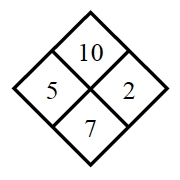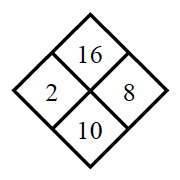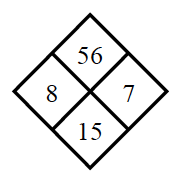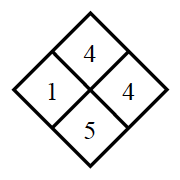Copy the Diamond Problems below and use the pattern you discovered to complete each of them.

Notice that the top number is the product of the middle numbers and the bottom number is the sum.
Now complete the diamonds in parts (a) through (d) using this pattern.

1.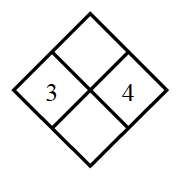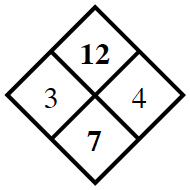1.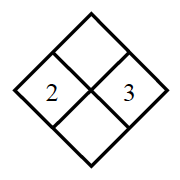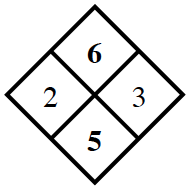1.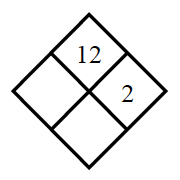$2\times?=12$

1.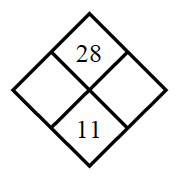What two numbers have a product of $28$ and a sum of $11$? Try factoring $28$ and check factors to see which two add up to $11$.